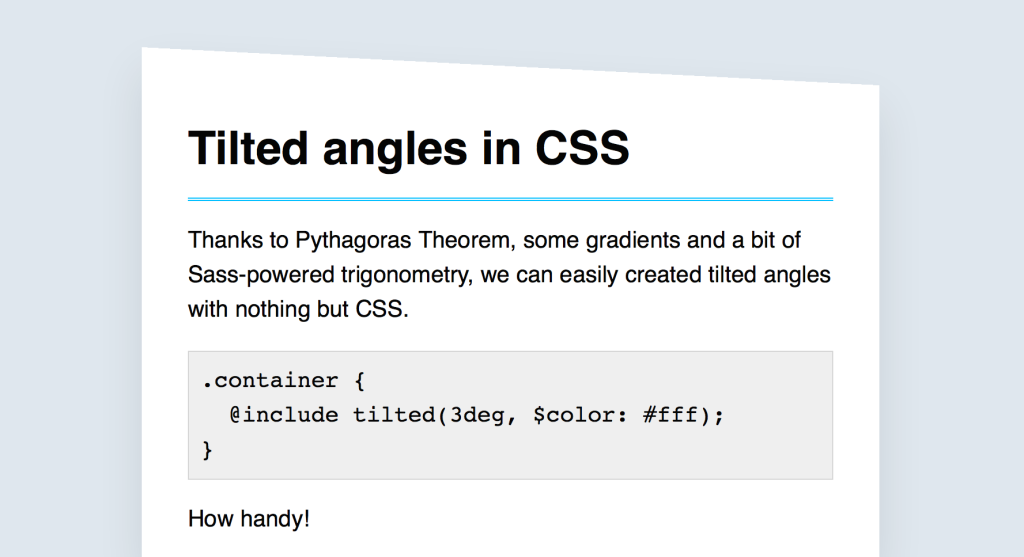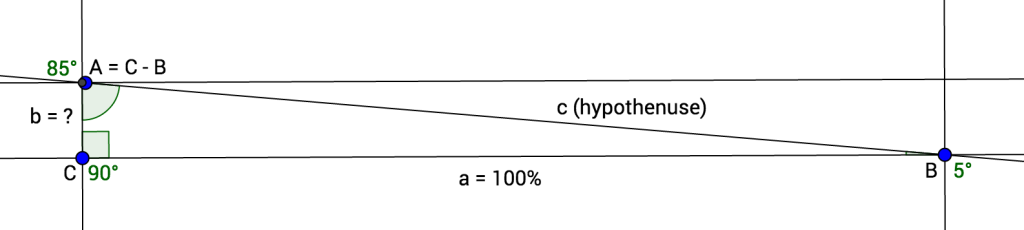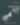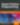# Tilted Angles in Sass

HTML & CSS

Share:

#### Free JavaScript Book!Write powerful, clean and maintainable JavaScript.

RRP \$11.95

Recently, I had to work on a website heavily using tilted angles as part of its design guidelines. By “tilted angles”, I mean these sections whose top or bottom edge is not completely horizontal and rather a bit inclined.There are quite a few ways to implement this. You could have base 64 encoded images applied as a background, but it makes it hard to customise (color, angle, etc.).

Another way would be to skew and/or rotate an absolutely positioned pseudo-element, but if there is one thing I don’t want to deal with, it’s the skew transform. God, no.

When using Sass, you could use the Angled Edges library that encodes dynamically generated SVG. It works super well, however it requires a fixed width and height expressed in pixels, which kind of bothered me.

I also really wanted to see if and how I could be able to implement this. I ended up with a solution that I am really proud of, even if it might be a bit over-engineered for simple scenarios.

## What’s the Idea?

My idea was to apply a half transparent, half solid gradient to an absolutely-positioned pseudo-element. The angle of the gradient defines the tilt angle.

``background-image: linear-gradient(\$angle, \$color 50%, transparent 50%);``

This is packaged in a mixin that applies the background color to the container, and generates a pseudo-element with the correct gradient based on the given angle. Simple enough. You’d use it like this:

``````.container {
@include tilted(\$angle: 3deg, \$color: rgb(255, 255, 255));
}``````

The main problem I faced with this was to figure which height the pseudo-element should have. At first, I made it an argument of the mixin, but I ended up going trial-and-error at every new angle to figure out what would be the best height for the pseudo. Not ideal.

And just when I was about to table flip the whole thing, I decided to leave the laptop away, took a pen and paper and started doodling to figure out the formula behind it. It took me a while (and a few Google searches) to remember trigonometry I learnt in high-school, but eventually I made it.

To avoid having to guess or come up with good approximations, the height of the pseudo-element is computed from the given angle. This is, of course, all done with a bit of Sass and a lot of geometry. Let’s go.

## Computing the Pseudo-element’s Height

Trust me when I’m telling you it won’t be too hard. The first thing we know is that we have a full-width pseudo-element. The gradient line will literally be the pseudo’s diagonal, so we end up with a rectangle triangle.

Let’s name it ABC, where `C` is the right angle, `B` is the known angle (`\$angle` argument) and `A` is therefore `C - B`. As shown on this diagram, we try to figure out what `b` is.To do that, we need to find the value of `c` (the gradient line, aka the hypothenuse), which is the length of `a` (the bottom side, 100%) divided by sine of the `A` angle (with `B = 5°`, `A` would be 85° for instance).

``c = a / sin(C - B)``

From there, we have to use Pythagoras theorem:

The square of the hypotenuse (the side opposite the right angle) is equal to the sum of the squares of the other two sides.

Therefore, the square of one of the other side equals the square of the hypothenuse minus the square of the third side. So the square of `b` equals the square of `c` minus the square of `a`.

``b² = c² - a²``

Finally, the length of `b` equals the squared root of the square of `c` minus the square of `a`.

``b = √(c² - a²)``

That’s it. We can now build a small Sass function computing the height of the pseudo-element based on a given angle.

``````@function get-tilted-height(\$angle) {
\$a: (100% / 1%);
\$A: (90deg - \$angle);
\$c: (\$a / sin(\$A));
\$b: sqrt(pow(\$c, 2) - pow(\$a, 2));

@return (abs(\$b) * 1%);
}``````

Note: the `pow()`, `sqrt()` and `sin()` functions can either come from Sassy-Math, Compass or custom sources.

## Building the Tilted Mixin

We’ve done the hardest part, trust me! The last thing to do is build the actual `tilted()` mixin. It accepts an angle and a color as argument and generates a pseudo-element.

``````@mixin tilted(\$angle, \$color) {
\$height: get-tilted-height(\$angle);

position: relative;
background-color: \$color;

&::before {
content: '';
position: absolute;
left: 0;
right: 0;
bottom: 100%;
background-image: linear-gradient(\$angle, \$color 50%, transparent 50%);
}
}``````

A few things to note here: the mixin applies `position: relative` to the container to define a position context for the pseudo-element. When using this mixin on absolute or fixed elements, it might be worth considering removing this declaration from the mixin.

The mixin applies the background color to the container itself as well as pseudo’s gradient, as they have to be synced anyway.

Finally, the pseudo-element’s height has to be conveyed through `padding-top` (or `padding-bottom` for that matter) instead of `height`. Since the height is expressed in percentages based on the parent’s width, we cannot rely on `height` (as it computes from the parent’s height).

## Final Thoughts and Going Further

For this article, I’ve chosen to go with a simple version of the mixin, which might lack flexibility and could, in theory, present the following problems:

• It is not possible to use it on an element already making use of its `::before` pseudo-element. This could be solved by adding an optional parameter to specify the pseudo-element, defaulting to `before`.
• It is not possible to display a tilted edge at the bottom of the container as `bottom: 0` is currently hard-coded in the mixin core. This could be solved by making it possible to pass an extra position to the mixin.

Also, my existing version uses Sass-based math functions as it was in a Jekyll project, not allowing me to extend the Sass layer. If using node-sass, you could easily pass these functions from JavaScript to Sass through Eyeglass or Sassport, which would be definitely much better.

I hope you liked it! If you can think of anything to improve it, please feel free to share in the comments. :)

See the Pen Tilted Angles in CSS by SitePoint (@SitePoint) on CodePen.### Hugo Giraudel

Non-binary accessibility & diversity advocate @n26, author, speaker. They/them.

#### New books out now!Learn how Git works, and how to use it to streamline your workflow!Google, Netflix and ILM are Python users. Maybe you should too?